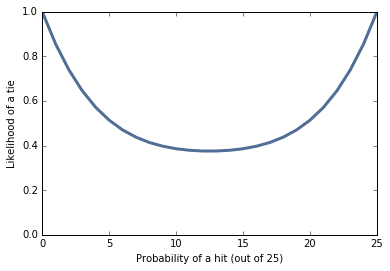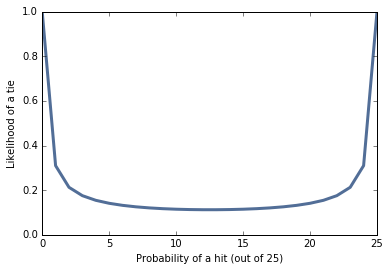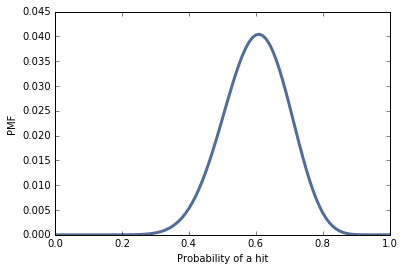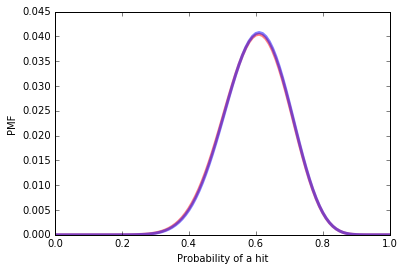## Tuesday, November 1, 2016

### The Skeet Shooting problem

Last week I posted the Skeet Shooting Problem:

At the 2016 Summer Olympics in the Women's Skeet event, Kim Rhode faced Wei Meng in the bronze medal match.  After 25 shots, they were tied, sending the match into sudden death.  In each round of sudden death, each competitor shoots at two targets.  In the first three rounds, Rhode and Wei hit the same number of targets.  Finally in the fourth round, Rhode hit more targets, so she won the bronze medal, making her the first Summer Olympian to win an individual medal at six consecutive summer games.  Based on this information, should we infer that Rhode and Wei had an unusually good or bad day?

As background information, you can assume that anyone in the Olympic final has about the same probability of hitting 13, 14, 15, or 16 out of 25 targets.

Now here's a solution:

As part of the likelihood function, I'll use binom.pmf, which computes the Binomial PMF.
In the following example, the probability of hitting k=10 targets in n=25 attempts, with probability p=13/15 of hitting each target, is about 8%.
In :
from scipy.stats import binom

k = 10
n = 25
p = 13/25
binom.pmf(k, n, p)

Out:
0.078169085615240511
The following function computes the likelihood of a tie (or no tie) after a given number of shots, n, given the hypothetical value of p.
It loops through the possible number of hits, k, from 0 to n and uses binom.pmf to compute the probability that each shooter hits k targets.
To get the probability that both shooters hit k targets, we square the result.
To get the total likelihood of the outcome, we add up the probability for each value of k.
In :
def likelihood(data, hypo):
"""Likelihood of data under hypo.

data: tuple of (number of shots, 'tie' or 'no tie')
hypo: hypothetical number of hits out of 25
"""
p = hypo / 25
n, outcome = data
like = sum([binom.pmf(k, n, p)**2 for k in range(n+1)])
return like if outcome=='tie' else 1-like

Let's see what that looks like for n=2
In :
data = 2, 'tie'

hypos = range(0, 26)
likes = [likelihood(data, hypo) for hypo in hypos]
thinkplot.Plot(hypos, likes)
thinkplot.Config(xlabel='Probability of a hit (out of 25)',
ylabel='Likelihood of a tie',
ylim=[0, 1])As we saw in the Sock Drawer problem and the Alien Blaster problem, the probability of a tie is highest for extreme values of p, and minimized when p=0.5.
The result is similar, but more extreme, when n=25:
In :
data = 25, 'tie'

hypos = range(0, 26)
likes = [likelihood(data, hypo) for hypo in hypos]
thinkplot.Plot(hypos, likes)
thinkplot.Config(xlabel='Probability of a hit (out of 25)',
ylabel='Likelihood of a tie',
ylim=[0, 1])In the range we care about (13 through 16 hits out of 25) this curve is pretty flat, which means that a tie after the round of 25 doesn't discriminate strongly among the hypotheses.
We could use this likelihood function to run the update, but just for purposes of demonstration, I'll use the Suite class from thinkbayes2:
In :
from thinkbayes2 import Suite

class Skeet(Suite):

def Likelihood(self, data, hypo):
"""Likelihood of data under hypo.

data: tuple of (number of shots, 'tie' or 'no tie')
hypo: hypothetical number of hits out of 25
"""
p = hypo / 25
n, outcome = data
like = sum([binom.pmf(k, n, p)**2 for k in range(n+1)])
return like if outcome=='tie' else 1-like

Now I'll create the prior.
In :
suite = Skeet([13, 14, 15, 16])
suite.Print()

13 0.25
14 0.25
15 0.25
16 0.25

The prior mean is 14.5.
In :
suite.Mean()

Out:
14.5
Here's the update after the round of 25.
In :
suite.Update((25, 'tie'))
suite.Print()

13 0.245787744767
14 0.247411480833
15 0.250757985003
16 0.256042789397

The higher values are a little more likely, but the effect is pretty small.
Interestingly, the rounds of n=2 provide more evidence in favor of the higher values of p.
In :
suite.Update((2, 'tie'))
suite.Print()

13 0.240111712333
14 0.243807684231
15 0.251622537592
16 0.264458065844

In :
suite.Update((2, 'tie'))
suite.Print()

13 0.234458172307
14 0.240145161205
15 0.252373188397
16 0.273023478091

In :
suite.Update((2, 'tie'))
suite.Print()

13 0.228830701632
14 0.236427057892
15 0.253007722855
16 0.28173451762

After three rounds of sudden death, we are more inclined to think that the shooters are having a good day.
The fourth round, which ends with no tie, provides a small amount of evidence in the other direction.
In :
suite.Update((2, 'no tie'))
suite.Print()

13 0.2323322732
14 0.23878553469
15 0.252684685857
16 0.276197506253

And the posterior mean, after all updates, is a little higher than 14.5, where we started.
In :
suite.Mean()

Out:
14.572747425162735
In summary, the outcome of this match, with four rounds of sudden death, provides weak evidence that the shooters were having a good day.
In general for this kind of contest, a tie is more likely if the probability of success is very high or low:
• In the Alien Blaster problem, the hypothetical value of p are all less than 50%, so a tie causes us to revise beliefs about p downward.
• In the Skeet Shooter problem, the hypothetical values are greater than 50%, so ties make us revise our estimates upward.

## Using a continuous prior¶

For simplicity, I started with a discrete prior with only 4 values. But the analysis we just did generalizes to priors with an arbitrary number of values. As an example I'll use a discrete approximation to a Beta distribution.
Suppose that among the population of Olympic skeet shooters, the distribution of p (the probability of hitting a target) is well-modeled by a Beta distribution with parameters (15, 10). Here's what that distribution looks like:
In :
from thinkbayes2 import Beta

beta = Beta(15, 10).MakePmf()
thinkplot.Pdf(beta)
thinkplot.Config(xlabel='Probability of a hit',
ylabel='PMF')We can use that distribution to intialize the prior:
In :
prior = Skeet(beta)
prior.Mean() * 25

Out:
14.999999999999991
And then perform the same updates:
In :
posterior = prior.Copy()
posterior.Update((25, 'tie'))
posterior.Update((2, 'tie'))
posterior.Update((2, 'tie'))
posterior.Update((2, 'tie'))
posterior.Update((2, 'no tie'))

Out:
0.088322129669977739
Here are the prior and posterior distributions. You can barely see the difference:
In :
thinkplot.Pdf(prior, color='red', alpha=0.5)
thinkplot.Pdf(posterior, color='blue', alpha=0.5)
thinkplot.Config(xlabel='Probability of a hit',
ylabel='PMF')And the posterior mean is only slightly higher.
In :
posterior.Mean() * 25

Out:
15.041026161545973